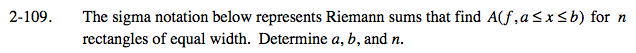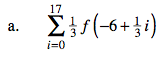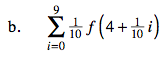### Home > CALC > Chapter Ch2 > Lesson 2.3.1 > Problem2-109

2-109.General form of left-endpoint Riemann Sum:

n represents number of rectangles
a represents starting value on the x-axis

$\Delta x\text{ represents width of each rectangle}:\frac{b-a}{n}$